Download the eSaral app and start learning from Kota's top IITians and doctors.

# NCERT Solutions for Class 11 Physics chapter 5 - Laws of Motion - Free PDF DownloadHey, are you a class 11 student and looking for ways to download NCERT Solutions for Class 11 Physics chapter 5 "Laws of Motion"? If yes. Then read this post till the end.

In this article, we have listed NCERT solution for class 11 Physics Chapter 5 in PDF that are prepared by Kota’s top IITian’s Faculties by keeping Simplicity in mind.

If you want to learn and understand class 11 Physics chapter 5 "Laws of Motion" in an easy way then you can use these solutions PDF.

NCERT Solutions helps students to Practice important concepts of subjects easily. Class 11 Physics solutions provide detailed explanations of all the NCERT questions that students can use to clear their doubts instantly.

If you want to score high in your class 11 Physics Exam then it is very important for you to have a good knowledge of all the important topics, so to learn and practice those topics you can use eSaral NCERT Solutions.

So, without wasting more time Let’s start.

### Download The PDF of NCERT Solutions for Class 11 Physics Chapter 5 "Laws of Motion"

Question 1. Answer the following questions regarding earth’s magnetism: (a) A vector needs three quantities for its specification. Name the three independent quantities conventionally used to specify the earth’s magnetic field.

Solution:
The three independent quantities that are conventionally used for specifying the earth's magnetic field are:
i. Magnetic declination,
ii. The angle of dip, and
iii. The horizontal component of the earth's magnetic field
(b) The angle of dip at a location in southern India is about 18º. Would you expect a greater or smaller dip angle in Britain?

Solution:
The angle of dip is the angle made with the horizontal by the Earth's magnetic field lines. It depends on how far the point is located from the North or the South pole. Since Britain is much closer to the North pole than the India. Hence the angle of dip will be greater in Britain.
(c) If you made a map of magnetic field lines at Melbourne in Australia, would the lines seem to go into the ground or come out of the ground?

Solution:
Earth’s magnetic field is hypothetically considered that a huge barn magnet is dipped inside the earth with its north pole near the geographic South Pole and its south pole near the geographic North Pole. The magnetic field lines emerge from a magnetic north pole (earth’s south pole) and terminate at a magnetic south pole (earth’s north pole). Hence, in a map earth's magnetic field lines at Melbourne in Australia would seem to come out of the ground.
(d) In which direction would a compass free to move in the vertical plane point to, if located right on the geomagnetic north or south pole?

Solution:
The earth’s magnetic field is exactly vertical at the geomagnetic poles (both north and south). The compass needle is free to rotate in the horizontal plane. Hence it may point in any direction.
(e) The earth's field, it is claimed, roughly approximates the field due to a dipole of magnetic moment $8 \times 10^{22} \mathrm{~J} \mathrm{~T}^{-1}$ located at its centre. Check the order of magnitude of this number in some way.

Solution:
Given:
The dipole moment of magnetic moment =$4 \pi \times 10^{-7} \mathrm{Tm} \mathrm{A}^{-1}$
We know the magnetic strength of a bar magnet is given as,$B=\frac{\mu_{0}}{4 \pi} \frac{M}{d^{3}}$
Where, $\mu_{0}=$ Permeability of free space $=4 \pi \times 10^{-7} \mathrm{~T} \mathrm{~m} \mathrm{~A}^{-1}$
$M=$ Magnetic moment
Now, the magnetic field at an equatorial point of earth’s dipole can be
written as,
$B=\frac{\mu_{0}}{4 \pi} \frac{M}{R^{3}}$\
$[R$ is the radius of the earth]
$\Rightarrow B=10^{-7} \times \frac{\left(8 \times 10^{22}\right)}{\left(6.4 \times 10^{6}\right)^{3}}=3.052 \times 10^{-5} \mathrm{~T}$
This value of the magnetic field is the same as Earth’ magnetic field strength at the equator.
(f) Geologists claim that besides the main magnetic N-S poles, there are several local poles on the earth’s surface oriented in different directions. How is such a thing possible at all?

Solution:
Yes, the geologist claims that besides the main magnetic N-S poles, there are several other local poles on the earth’s surface oriented in different directions is true. On the surface of the earth, there are many places of magnetising mineral deposits. These deposits create their own magnetic NS poles, which can interfere with the earth’s magnetic field.

Question 2. Answer the following questions:
(a) The earth’s magnetic field varies from point to point in space. Does it also change with time? If so, on what time scale does it change appreciably?

Solution:
Earth's magnetic field does vary from point to point in space and also with time. This change in the magnetic field can happen daily, annually or with a period of the order of about 1000 year and also irregularly due to magnetic storms etc. Noticeable changes occur over a large period of time(few hundred years) and variations are there even in small durations of time (few years) which cannot be ignored.
(b) The earth’s core is known to contain iron. Yet geologists do not regard this as a source of the earth’s magnetism. Why?

Solution:
Earth’s core contains molten iron, which is not ferromagnetic. Hence, it cannot be considered as a source of earth’s magnetism.
(c) The charged currents in the outer conducting regions of the earth’s core are thought to be responsible for earth’s magnetism. What might be the ‘battery’ (i.e., the source of energy) to sustain these currents?

Solution:
The charged currents in the Earth's core's exterior conductive areas are believed to be accountable for the magnetism of Earth. However, the nprecise mode of these circulating currents and the energy needed to sustain them are not very well understood and it is an area of research.
(d) The earth may have even reversed the direction of its field several times during its history of 4 to 5 billion years. How can geologists know about the earth’s field in such distant past?

Solution:
The magnetic field of earth reversed its direction several times during its history. These change in magnetic fields got weakly recorded in rocks during their solidification. So, we can get clues about the geomagnetic history from the analysis of this rock magnetism

(e) The earth’s field departs from its dipole shape substantially at large distances (greater than about 30,000 km). What agencies may be nresponsible for this distortion?

Solution:
Due to the presence of the ionosphere, the Earth's magnetic fielddeparts from its dipole at large distances (higher than about 30,000 km). In the ionosphere, the earth’s magnetic field gets modified because of then magnetic field produced by the motion of the ions.
(f) Interstellar space has an extremely weak magnetic field of the order of $10^{-}$ 12 T. Can such a weak field be of any significant consequence? Explain.

Solution:
At very-very large distances like interstellar distances, the small fields can significantly affect the charged particles like that of cosmic rays. For small distances, the deflections are not noticeable for small fields, but at very large distances the deflections are significant.

Question 3. A short bar magnet placed with its axis at $30^{\circ}$ with a uniform external magnetic field of $0.25 \mathrm{~T}$ experiences a torque of magnitude equal to $4.5 \times 10^{-2} \mathrm{~N} \mathrm{~m}$. What is the magnitude of the magnetic moment of the magnet?

Solution:
Given:
Magnetic field strength, $B=0.25 \mathrm{~T}$
Torque on the bar magnet, $T=4.5 \times 10^{-2} \mathrm{~N} \mathrm{~m}$
The angle between the short bar magnet and the external magnetic field, $\theta=30^{\circ}$
The relation between Torque and magnetic moment (M)
$T=M B \sin \theta$
$\Rightarrow \mathrm{M}=\frac{\mathrm{T}}{\mathrm{B} \sin \theta}=\frac{4.5 \times 10^{-2}}{0.25 \sin 30}=0.36 \mathrm{~J} \mathrm{~T}^{-1}$

Question 4. A short bar magnet of magnetic moment $\mathrm{m}=0.32 \mathrm{~J} \mathrm{~T}^{-1}$ is placed in a uniform magnetic field of $0.15 \mathrm{~T}$. If the bar is free to rotate in the plane of the field, which orientation would correspond to its (a) stable, and (b) unstable equilibrium? What is the potential energy of the magnet in each case?

Solution:
Given:
The magnetic moment of the bar magnet, $\mathrm{M}=0.32 \mathrm{~J} \mathrm{~T}^{-1}$
External magnetic field, $\mathrm{B}=0.15 \mathrm{~T}$
(a) When the bar magnet and the magnetic field are in same direction or the angle between them is $\theta=0^{\circ} .$ Then the magnet is in stable equilibrium.
The potential energy of the system
$=-\mathrm{MB} \cos \theta$
$=-0.32 \times 0.15 \cos 0^{\circ}$
$=-4.8 \times 10^{-2} \mathrm{~J}$
(b) When the bar magnet makes $180^{\circ}$ angle to the magnetic field. It is in an unstable equilibrium.
The potential energy of the system
$=-\mathrm{MB} \cos \theta$
$=-0.32 \times 0.15 \cos 180^{\circ}$
$=4.8 \times 10^{-2} \mathrm{~J}$

Question 5. A closely wound solenoid of 800 turns and area of cross section $2.5 \times 10^{-4} \mathrm{~m}^{2}$ carries a current of $3.0 \mathrm{~A}$. Explain the sense in which the solenoid acts like a bar magnet. What is its associated magnetic moment?

Solution:
Given:
Number of turns in the solenoid, $n=800$
Area of cross-section, $A=2.5 \times 10^{-4} \mathrm{~m}^{2}$
Current in the solenoid, $I=3.0 \mathrm{~A}$
The magnetic field produced by a current-carrying solenoid is along its axis. Hence it behaves like a bar magnet.
The magnetic moment associated with the current-carrying solenoid is
$M=n I A$
$=800 \times 3 \times 2.5 \times 10^{-4}$
$=0.6 \mathrm{~J} \mathrm{~T}^{-1}$
Question 6. If the solenoid in Exercise $5.5$ is free to turn about the vertical direction and a uniform horizontal magnetic field of $0.25 \mathrm{~T}$ is applied, what is the magnitude of torque on the solenoid when its axis makes an angle of $30^{\circ}$ with the direction of applied field?

Solution:
Given:
Magnetic field strength, $B=0.25 \mathrm{~T}$
Magnetic moment, $M=0.6 \mathrm{~J} \mathrm{~T}^{-1}$
The angle between the solenoid axis and the magnetic field direction is $30^{\circ}$.
Now, the torque acting on the solenoid is
$\mathrm{T}=\mathrm{MB} \sin \theta$
$=0.6 \times 0.25 \sin 30^{\circ}$
$=7.5 \times 10^{-2} \mathrm{~N}-\mathrm{m}$

Question 7. A bar magnet of magnetic moment $1.5 \mathrm{~J} \mathrm{~T}^{-1}$ lies aligned with the direction of a uniform magnetic field of $0.22 \mathrm{~T}$. (a) What is the amount of work required by an external torque to turn the magnet so as to align its magnetic moment: (i) normal to the field direction, (ii) opposite to the field direction? (b) What is the torque on the magnet in cases (i) and (ii)?

Solution:
(a) Given:
Magnetic moment, $\mathrm{M}=1.5 \mathrm{~J} \mathrm{~T}^{-1}$
Magnetic field strength, $\mathrm{B}=0.22 \mathrm{~T}$
(i) The initial angle between the axis and the magnetic field, $\theta_{1}=0^{\circ}$
The final angle between the axis and the magnetic field, $\theta_{2}=90^{\circ}$
The work needed to make the magnetic moment normal to the direction of the magnetic field is given as:
$\mathrm{W}=-\mathrm{MB}\left(\cos \theta_{2}-\cos \theta_{1}\right)$
$=-1.5 \times 0.22\left(\cos 90^{\circ}-\cos 0^{\circ}\right)$
$=-0.33(0-1)=0.33 \mathrm{~J}$
(ii) The initial angle between the axis and the magnetic field, $\theta_{1}=0^{\circ}$
The final angle between the axis and the magnetic field, $\theta_{2}=180^{\circ}$
The work required to make the magnetic moment opposite to the direction of the magnetic field is given as:
$\mathrm{W}=-\mathrm{MB}\left(\cos \theta_{2}-\cos \theta_{1}\right)$
$=-1.5 \times 0.22\left(\cos 180^{\circ}-\cos 0^{\circ}\right)$
$=-0.33(-1-1)$
$=0.66 \mathrm{~J}$
(b) For case (i): $\theta=90^{\circ}$
$\therefore$ Torque,
$\mathrm{T}=\mathrm{MB} \sin \theta$
$=1.5 \times 0.22 \sin 90^{\circ}$
$=0.33 \mathrm{~J}$
For case (ii): $\theta=180^{\circ}$
$\therefore$ Torque,
$\mathrm{T}=\mathrm{MB} \sin \theta$
$=\mathrm{MB} \sin 180^{\circ}=0$

Questiom 8. A closely wound solenoid of 2000 turns and area of cross-section $1.6 \times 10^{-4} \mathrm{~m}^{2}$, carrying a current of $4.0 \mathrm{~A}$, is suspended through its centre allowing it to turn in a horizontal plane.
(a) What is the magnetic moment associated with the solenoid?
(b) What is the force and torque on the solenoid if a uniform horizontal magnetic field of $7.5 \times 10^{-2} \mathrm{~T}$ is set up at an angle of $30^{\circ}$ with the axis of the solenoid?

Solution:
Given:
Number of turns on the solenoid, 𝑛𝑛 = 2000
Area of the cross-section of the solenoid, $\mathrm{A}=1.6 \times 10^{-4} \mathrm{~m}^{2}$
Current in the solenoid, $I=4 \mathrm{~A}$
(a) The magnetic moment on the solenoid axis
$\mathrm{M}=\mathrm{nAI}$
$=2000 \times 1.6 \times 10^{-4} \times 4$
$=1.28 \mathrm{~A} \mathrm{~m}^{2}$
(b) $\quad$ Magnetic field, $\mathrm{B}=7.5 \times 10^{-2} \mathrm{~T}$
Angle between the solenoid axis and the magnetic field, $\theta=30^{\circ}$
Torque, $\mathrm{T}=\mathrm{MB} \sin \theta$
$=1.28 \times 7.5 \times 10^{-2} \sin 30^{\circ}$
$=4.8 \times 10^{-2} \mathrm{~N}-\mathrm{m}$

Question 9. A circular coil of 16 turns and radius $10 \mathrm{~cm}$ carrying a current of $0.75 \mathrm{~A}$ rests with its plane normal to an external field of magnitude $5.0 \times 10^{-2} \mathrm{~T}$. The coil is free to turn about an axis in its plane perpendicular to the field direction. When the coil is turned slightly and released, it oscillates about its stable equilibrium with a frequency of $2.0 \mathrm{~s}^{-1} .$ What is the moment of inertia of the coil about its axis of rotation?

Solution:
Given:
Number of turns in the circular coil, $N=16$
Radius of the coil, $\mathrm{r}=10 \mathrm{~cm}=0.1 \mathrm{~m}$
Cross-section of the coil, $A=\pi r^{2}=\pi \times(0.1)^{2} \mathrm{~m}^{2}$
Current in the coil, $I=0.75 \mathrm{~A}$
Magnetic field strength, $B=5.0 \times 10^{-2} \mathrm{~T}$
Frequency of oscillations of the coil, $f=2.0 \mathrm{~s}^{-1}$
$\therefore$ Magnetic moment, $M=$ NIA
$=16 \times 0.75 \times \pi \times(0.1)^{2}$
$=0.377 \mathrm{~J} \mathrm{~T}^{-1}$
Frequency is given by the relation, $f=\frac{1}{2 \pi} \sqrt{\frac{M B}{I}}$
Where, $\mathrm{I}=$ Moment of inertia of the coil
$\Rightarrow I=\frac{M B}{4 \pi^{2} f^{2}}=\frac{0.377 \times 5 \times 10^{-2}}{4 \times \pi^{2} \times(2)^{2}}=1.19 \times 10^{-4} \mathrm{~kg} \mathrm{~m}^{2}$
Hence, the moment of inertia of the coil about its axis of rotation is
$1.19 \times 10^{-4} \mathrm{~kg} \mathrm{~m}^{2}$

Question 10. A magnetic needle free to rotate in a vertical plane parallel to the magnetic meridian has its north tip pointing down at $22^{\circ}$ with the horizontal. The horizontal component of the earth's magnetic field at the place is known to be $0.35 \mathrm{G}$. Determine the magnitude of the earth's magnetic field at the place.

Solution:
Given:
The horizontal component of the magnetic field of the earth is, $\mathrm{B}_{\mathrm{H}}=0.35 \mathrm{G}$
The angle of dip $=22^{\circ}$
Earth's magnetic field strength $=\mathrm{B}$
We can relate $\mathrm{B}$ and $\mathrm{B}_{\mathrm{H}}$ as:
$\mathrm{B}_{\mathrm{H}}=\mathrm{B} \cos \theta$
$\Rightarrow \mathrm{B}=\frac{\mathrm{B}_{\mathrm{H}}}{\cos \theta}=\frac{0.35}{\cos 22^{\circ}}=0.377 \mathrm{G}$

Question 11. At a certain location in Africa, a compass points $12^{\circ}$ west of the geographic north. The north tip of the magnetic needle of a dip circle placed in the plane of magnetic meridian points $60^{\circ}$ above the horizontal. The horizontal component of the earth's field is measured to be $0.16 \mathrm{G}$. Specify the direction and magnitude of the earth's field at the location.
Solution:
Given:
The angle of declination, $\delta=12^{\circ}$
The angle of dip, $\theta=60^{\circ}$
The horizontal component of the magnetic field of the earth is, $B_{H}=0.16 \mathrm{G}$
Earth's magnetic field at the given location $=B$
We can relate $\mathrm{B}$ and $\mathrm{B}_{\mathrm{H}}$ as:
$\mathrm{B}_{\mathrm{H}}=\mathrm{B} \cos \theta$
$\Rightarrow \mathrm{B}=\frac{\mathrm{B}_{\mathrm{H}}}{\cos \theta}=\frac{0.16}{\cos 60^{\circ}}=0.32 \mathrm{G}$
Earth's magnetic field lies in the vertical plane, $12^{\circ}$ West of the geographic meridian, making an angle of $60^{\circ}$ (upward) with the horizontal direction. Its magnitude is $0.32 \mathrm{G}$.

Question 12. A short bar magnet has a magnetic moment of $0.48 \mathrm{~J} \mathrm{~T}^{-1}$. Give the direction and magnitude of the magnetic field produced by the magnet at a distance of $10 \mathrm{~cm}$ from the centre of the magnet on (a) the axis, (b) the equatorial lines (normal bisector) of the magnet.

Solution:
Given:
The magnetic moment of the bar magnet, $M=0.48 \mathrm{~J} \mathrm{~T}^{-1}$
(a) Along the axis:
Distance, $r=10 \mathrm{~cm}=0.1 \mathrm{~m}$
The magnetic field at distance $r$, from the centre of the magnet on the axis, is given by the relation:
$B=\frac{\mu_{0}}{4 \pi} \frac{2 M}{r^{3}}$
Where, $\mu_{0}=$ Permeability of free space $=4 \pi \times 10^{-7} \mathrm{~T} \mathrm{~m} \mathrm{~A}^{-1}$
$\Rightarrow B=\frac{4 \pi \times 10^{-7}}{4 \pi} \times \frac{2 \times 0.48}{(0.1)^{3}}=0.96 \times 10^{-4} \mathrm{~T}$ The direction of magnetic field along the axis of a bar magnet could be towards magnet (south pole) or away from the magnet (north pole).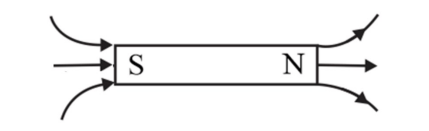(b) Along the equatorial line:
The magnetic field at a distance $r$ on the equatorial line of the magnet is given as:
$B=\frac{\mu_{0}}{4 \pi} \frac{M}{r^{3}}$
$\Rightarrow B=\frac{4 \pi \times 10^{-7}}{4 \pi} \times \frac{0.48}{(0.1)^{3}}=0.48 \times 10^{-4} \mathrm{~T}$
At equatorial line (or normal bisector) the magnetic field is parallel to the magnet and directed from north pole to south pole of the magnet.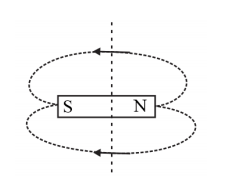Question 13. A short bar magnet placed in a horizontal plane has its axis aligned along the magnetic north-south direction. Null points are found on the axis of the magnet at $14 \mathrm{~cm}$ from the centre of the magnet. The earth's magnetic field at the place is $0.36$ $\mathrm{G}$ and the angle of dip is zero. What is the total magnetic field on the normal bisector of the magnet at the same distance as the -point (i.e., $14 \mathrm{~cm}$ ) from the centre of the magnet? (At points, field due to a magnet is equal and opposite to the horizontal component of earth's magnetic field.)

Solution:
The above situation can be represented as follows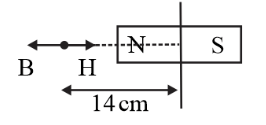Earth's magnetic field at the given place, $H=0.36 G$
$[\therefore$ The angle of dip is zero $]$
The magnetic field at point (distance $r)$, on the axis of the magnet, can be given as:
$B=\frac{\mu_{0}}{4 \pi} \frac{2 M}{r^{3}}=H$
Where, $\mu_{0}=$ Permeability of free space
$M=$ Magnetic moment
The magnetic field at the same distance $r$, on the equatorial line of the magnet, is given as:
$B_{1}=\frac{\mu_{0}}{4 \pi} \frac{M}{r^{3}}=\frac{H}{2}$
At equatorial line the magnetic field of magnet and earth's magnetic field will get added as shown in figure.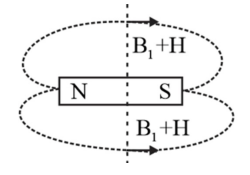The total magnetic field, $B=B_{1}+H$
$\Rightarrow B=H+\frac{H}{2}=\frac{3 H}{2}=0.54 \mathrm{G}$

Question 14. If the bar magnet in exercise $5.13$ is turned around by $180^{\circ}$, where will the new points be located?

Solution:
The magnetic field along the axis of the magnet at a distance $r_{1}=14 \mathrm{~cm}$, can be written as:
$B=\frac{\mu_{0}}{4 \pi} \frac{2 M}{r_{1}^{3}} \quad \ldots \ldots(1)$
If the bar magnet is turned through $180^{\circ}$, then the neutral point will lie on the equatorial line as shown in figure.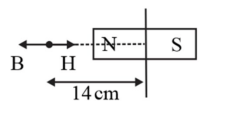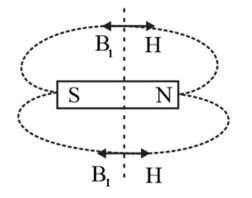Hence, the magnetic field at a distance $r_{2}$, on the equatorial line of the magnet can be written as:
$B=\frac{\mu_{0}}{4 \pi} \frac{M}{r_{2}^{3}} \quad \ldots \ldots$ (2)
Equating equations (1) and (2), we get:
$\frac{2}{r_{1}^{3}}=\frac{1}{r_{2}^{3}}$
$\Rightarrow r_{2}=r_{1}\left(\frac{1}{2}\right)^{1 / 3}=14 \times 0.794=11.1 \mathrm{~cm}$
The new points will be located $11.1 \mathrm{~cm}$ on the normal bisector.

Question 15. A short bar magnet of magnetic moment $5.25 \times 10^{-2} \mathrm{~J} \mathrm{~T}^{-1}$ is placed with its axis perpendicular to the earth's field direction. At what distance from the centre of the magnet, the resultant field is inclined at $45^{\circ}$ with earth's field on (a) its normal bisector and (b) its axis. Magnitude of the earth's field at the place is given to be $0.42 \mathrm{G}$. Ignore the length of the magnet in comparison to the distances involved.

Solution:
Given:
The magnetic moment of the bar magnet, $M=5.25 \times 10^{-2} \mathrm{~J} \mathrm{~T}^{-1}$
Magnitude of earth's magnetic field, $H=0.42 G=0.42 \times 10^{-4} \mathrm{~T}$
(a) The magnetic field at a distance $R$ from the centre of the magnet on the normal bisector is given by the relation:
$B=\frac{\mu_{0}}{4 \pi} \frac{M}{d^{3}}$
Where, $\mu_{0}=$ Permeability of free space $=4 \pi \times 10^{-7} \mathrm{~T} \mathrm{~m} \mathrm{~A}^{-1}$
When the resultant field is inclined at $45^{\circ}$ with the earth's field, $\therefore B=H$
$\Rightarrow \frac{\mu_{0}}{4 \pi} \frac{M}{R^{3}}=H=0.42 \times 10^{-4}$
$\Rightarrow R^{3}=\frac{\mu_{0} M}{4 \pi \times 0.42 \times 10^{-4}}=1.25 \times 10^{-4}$
$\Rightarrow R=0.05 \mathrm{~m}=5 \mathrm{~cm}$(b) The magnetic field at a distanced $R^{\prime}$ from the centre of the magnet on its axis is given as:
$B^{\prime}=\frac{\mu_{0}}{4 \pi} \frac{2 M}{R^{3}}$
The resultant field is inclined at $45^{\circ}$ with the earth's field.
$\therefore B^{\prime}=H$
$\Rightarrow \frac{\mu_{0}}{4 \pi} \frac{2 M}{R^{3}}=H=0.42 \times 10^{-4}$
$\Rightarrow R^{\prime 3}=\frac{\mu_{0}(2 M)}{4 \pi \times 0.42 \times 10^{-4}}=2.5 \times 10^{-4}$
$\Rightarrow R^{\prime}=0.063 \mathrm{~m}=6.3 \mathrm{~cm}$
(b) The magnetic field at a distanced $R^{\prime}$ from the centre of the magnet on its axis is given as:
$B^{\prime}=\frac{\mu_{0}}{4 \pi} \frac{2 M}{R^{3}}$
The resultant field is inclined at $45^{\circ}$ with the earth's field.
$\therefore B^{\prime}=H$
$\Rightarrow \frac{\mu_{0}}{4 \pi} \frac{2 M}{R^{3}}=H=0.42 \times 10^{-4}$
$\Rightarrow R^{\prime 3}=\frac{\mu_{0}(2 M)}{4 \pi \times 0.42 \times 10^{-4}}=2.5 \times 10^{-4}$
$\Rightarrow R^{\prime}=0.063 \mathrm{~m}=6.3 \mathrm{~cm}$

Question 16. Answer the following questions:
(a) Why does a paramagnetic sample display greater magnetisation (for the same magnetising field) when cooled?

Solution:

The dipole alignment gets interrupted at elevated temperatures because of the random motion of the molecules. On cooling, this disruption is reduced. Hence, a paramagnetic sample displays greater magnetisation when cooled.
(b) Why is diamagnetism, in contrast, almost independent of temperature?

Solution:

In diamagnetic substances, resultant magnetic moment in an atom is zero. Hence, the internal motion of the atoms does not affect the diamagnetism of a material. Thus, diamagnetism is almost independent of temperature.
(c) If a toroid uses bismuth for its core, will the field in the core be (slightly) greater or (slightly) less than when the core is empty?

Solution:
Bismuth is a diamagnetic substance. Hence, a toroid with a bismuth core has a magnetic field slightly greater than a toroid whose core is empty.
(d) Is the permeability of a ferromagnetic material independent of the magnetic field? If not, is it more for lower or higher fields?

Solution:
The permeability of ferromagnetic materials dependents of the applied magnetic field. It is greater for a lower field and lesser for the higher field.
(e) Magnetic field lines are always nearly normal to the surface of a ferromagnet at every point. (This fact is analogous to the static electric field lines being normal to the surface of a conductor at every point.) Why?

Solution:
A ferromagnetic material's permeability is always greater than one. Hence, magnetic field lines are nearly normal to the surface of such materials at every point.
(f) Would the maximum possible magnetisation of a paramagnetic sample be of the same order of magnitude as the magnetisation of a ferromagnet?

Solution:
The maximum possible magnetisation of a paramagnetic sample can be of the same order of magnitude as the magnetisation of a ferromagnet. This requires high magnetising fields for saturation.

(a) Explain qualitatively on the basis of domain picture the irreversibility in the magnetisation curve of a ferromagnet.

Solution:
The hysteresis curve $(B-H$ curve) of a ferromagnetic material is shown in the figure.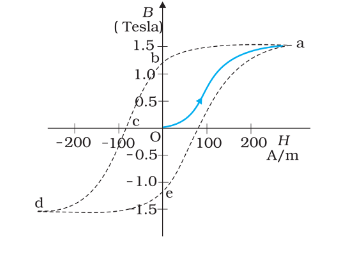It can be observed from the given curve that magnetisation persists even when the external field is removed. This reflects the irreversibility of a ferromagnet.
(b) The hysteresis loop of a soft iron piece has a much smaller area than that of a carbon steel piece. If the material is to go through repeated cycles of magnetisation, which piece will dissipate greater heat energy?

Solution:
The dissipated heat energy is in repeated cycles of magnetisation is directly proportional to the area of a hysteresis loop. A carbon steel piece has a greater hysteresis curve area than a soft iron piece. Hence, the carbon steel piece will dissipate greater heat energy compared to the soft iron piece.
(c) 'A system displaying a hysteresis loop such as a ferromagnet, is a device for storing memory?' Explain the meaning of this statement.

Solution:
The word hysteresis means lagging behind. When we see at the hysteresis curve of a material for a given value of $\mathrm{H}, \mathrm{B}$ is not unique but depends on the previous history of the sample. This can help for storing memory in devices.
(d) What kind of ferromagnetic material is used for coating magnetic tapes in a cassette player, or for building 'memory stores' in a modern computer?

Solution:
The ferromagnetic material is used for coating magnetic tapes in a cassette player, or for building 'memory stores' in a modern computer is called ferrite. It is a ceramic material made by mixing and firing large proportions of iron $(\mathrm{III})$ oxide $\left(\mathrm{Fe}_{2} \mathrm{O}_{3}\right.$, rust) blended with small proportions of one or more additional metallic elements, such as barium, manganese, nickel, and zinc.
(e) A certain region of space is to be shielded from magnetic fields. Suggest a method.

Solution:
To shield a certain region of space from magnetic fields we can surround the space by soft iron. As the magnetic field lines will be drawn into the soft iron and the enclosed region will become free of the magnetic field.

Question 18. A long straight horizontal cable carries a current of $2.5 \mathrm{~A}$ in the direction $10^{\circ}$ south of west to $10^{\circ}$ north of east. The magnetic meridian of the place happens to be $10^{\circ}$ west of the geographic meridian. The earth's magnetic field at the location is $0.33$ G, and the angle of dip is zero. Locate the line of neutral points (ignore the thickness of the cable). (At neutral points, magnetic field due to a current-carrying cable is equal and opposite to the horizontal component of earth's magnetic field.)

Solution:
Given:
Current in the wire, $I=2.5 \mathrm{~A}$
The angle of dip at the given location on earth, $\delta=0^{\circ}$
Earth's magnetic field, $B=0.33 G=0.33 \times 10^{-4} \mathrm{~T}$
The horizontal component of the earth's magnetic field is given as:
$B_{H}=B \cos \delta=0.33 \times 10^{-4} \times \cos 0^{\circ}=0.33 \times 10^{-4} \mathrm{~T}$
The orientation of cable can be understood by the following figure.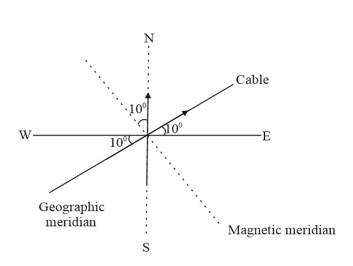The magnetic field at the neutral point at a distance $R$ from the cable is given by the relation:
$B^{\prime}=\frac{\mu_{0}}{4 \pi} \times \frac{2 I}{R}$
For the neutral point,
$B^{\prime}=\frac{\mu_{0}}{4 \pi} \times \frac{2 I}{R}=B_{H}$
Here, $I=2.5 \mathrm{~A}$ and $B_{H}=0.33 \times 10^{-4} \mathrm{~T}$
$\Rightarrow 0.33 \times 10^{-4}=10^{-7} \times \frac{2 \times 2.5}{R}$
$\Rightarrow R=\frac{5 \times 10^{-7}}{0.33 \times 10^{-4}}=15 \times 10^{-3} \mathrm{~m}$
or $R=1.5 \mathrm{~cm}$
Hence, a set of neutral points parallel to and above the cable are located at a normal distance of $1.5 \mathrm{~cm}$.

Question 19. A telephone cable at a place has four long straight horizontal wires carrying a current of $1.0 \mathrm{~A}$ in the same direction east to west. The earth's magnetic field at the place is $0.39 \mathrm{G}$, and the angle of dip is $35^{\circ}$. The magnetic declination is nearly zero. What are the resultant magnetic fields at points $4.0 \mathrm{~cm}$ below the cable?

Solution:
Given:
Current in the wire, $I=0.35 \mathrm{~A}$
The angle of dip, $\delta=35^{\circ}$
Earth's magnetic field, $\mathrm{B}=0.39 G=0.39 \times 10^{-4} \mathrm{~T}$
Magnetic field due to an infinitely long current carrying wire is
$\mathrm{B}_{1}=\frac{\mu_{0}}{4 \pi} \times \frac{2 I}{R}=10^{-7} \times \frac{(2 \times 1)}{0.04}=5 \times 10^{-6}$
The total magnetic field, $\mathrm{B}^{\prime}=4 \times \mathrm{B}_{1}=2 \times 10^{-5} \mathrm{~T}$
The magnetic field can be represented as follows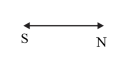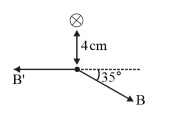The horizontal component of the earth magnetic field
$B_{H}=B \cos \delta=0.39 \times 10^{-4} \times \cos 35^{\circ}=3.19 \times 10^{-5} \mathrm{~T}$
The vertical component of the earth magnetic field is
$B_{V}=B \sin \delta=0.39 \times 10^{-4} \times \sin 35^{\circ}=2.2 \times 10^{-5} \mathrm{~T}$
At the point below the cable is
$B_{H}^{\prime}=B_{H}-B_{\text {total }}=3.19 \times 10^{-5}-2 \times 10^{-5}=1.19 \times 10^{-5} \mathrm{~T}$
The resultant magnetic field is
$\sqrt{B_{H}^{\prime 2}+B_{V}^{2}}=\sqrt{\left(1.19 \times 10^{-5}\right)^{2}+\left(2.2 \times 10^{-5}\right)^{2}}=2.5 \times 10^{-5} \mathrm{~T}$

Question 20. A compass needle free to turn in a horizontal plane is placed at the centre of circular coil of 30 turns and radius $12 \mathrm{~cm}$. The coil is in a vertical plane making an angle of $45^{\circ}$ with the magnetic meridian. When the current in the coil is $0.35 \mathrm{~A}$, the needle points west to east. (a) Determine the horizontal component of the earth's magnetic field at the location. (b) The current in the coil is reversed, and the coil is rotated about its vertical axis by an angle of $90^{\circ}$ in the anticlockwise sense looking from above. Predict the direction of the needle. Take the magnetic declination at the places to be zero.

Solution:
Given:
Number of turns in the circular coil, $N=30$
Radius of the circular coil, $r=12 \mathrm{~cm}=0.12 \mathrm{~m}$
Current in the coil, $I=0.35 \mathrm{~A}$
The angle of dip, $\delta=45^{\circ}$
(a) The magnetic field at the centre of a circular loop of radius $r$ and current I is given as:
$B=\frac{\mu_{0} I}{2 r}$
$\Rightarrow B=\frac{4 \pi \times 10^{-7} \times 30 \times 0.35}{2 \times 0.12}=5.5 \times 10^{-5} \mathrm{~T}$
The compass needle points from West to East. Hence, the horizontal component of earth's magnetic field is given as:
$B_{H}=B \cos \delta=5.5 \times 10^{-5} \times \sin 45^{\circ}=3.89 \times 10^{-5} \mathrm{~T}$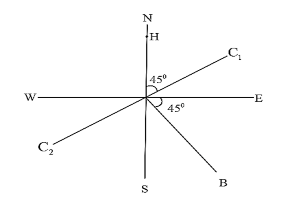(b) When the current in the coil is reversed and the coil is rotated about its vertical axis by an angle of $90^{\circ}$, the needle will reverse its original direction. In this case, the needle will point from East to West.

Question 21. A magnetic dipole is under the influence of two magnetic fields. The angle between the field directions is $60^{\circ}$, and one of the fields has a magnitude of $1.2 \times 10^{-2} \mathrm{~T}$. If the dipole comes to stable equilibrium at an angle of $15^{\circ}$ with this field, what is the magnitude of the other field?

Solution:
Given:
The magnitude of one of the magnetic fields, $B_{1}=1.2 \times 10^{-2} \mathrm{~T}$
The magnitude of the other magnetic field $=B_{2}$
The angle between the two fields, $\theta=60^{\circ}$
At stable equilibrium, the angle between the dipole and field $B_{1}, \theta_{1}=15^{\circ}$
The angle between the dipole and field $B_{2}, \theta_{2}=\theta-\theta_{1}=60^{\circ}-15^{\circ}=45^{\circ}$
At rotational equilibrium, the torques between both the fields must balance each other.
$\therefore$ The torque due to field $B_{1}=$ Torque due to field $B_{2}$
$M B_{1} \sin \theta_{1}=M B_{2} \sin \theta_{2}$
$\Rightarrow B_{2}=\frac{B_{1} \sin \theta_{1}}{\sin \theta_{2}}=1.2 \times 10^{-2} \times \frac{\sin 15^{\circ}}{\sin 45^{\circ}}=4.4 \times 10^{-3} \mathrm{~T}$
Hence, the magnitude of the other field is $4.4 \times 10^{-3} \mathrm{~T}$.

Question 22. A monoenergetic (18 keV) electron beam initially in the horizontal direction is subjected to a horizontal magnetic field of $0.04 \mathrm{G}$ normal to the initial direction. Estimate the up or down deflection of the beam over a distance of $30 \mathrm{~cm}\left(m_{e}=\right.$ $9.11 \times 10^{-31} \mathrm{~kg}$ ). [Note: Data in this exercise are so chosen that the answer will give you an idea of the effect of earth's magnetic field on the motion of the electron beam from the electron gun to the screen in a TV set.]

Solution:
Given:
Energy of an electron beam, $E=18 \mathrm{keV}=18 \times 10^{3} \mathrm{eV}$
$E=18 \times 10^{3} \times 1.6 \times 10^{-19} \mathrm{~J}$
The magnetic field, $B=0.04 \mathrm{G}$
Mass of an electron, $m_{e}=9.11 \times 10^{-19} \mathrm{~kg}$
Distance up to which the electron beam travels, $d=30 \mathrm{~cm}=0.3 \mathrm{~m}$
We can write the kinetic energy of the electron beam as:
$E=\frac{1}{2} m_{e} v^{2}$
$\Rightarrow v=\sqrt{\frac{2 E}{m_{e}}}=\sqrt{\frac{2 \times 18 \times 10^{3} \times 1.6 \times 10^{-19}}{9.11 \times 10^{-31}}}=7.96 \times 10^{7} \mathrm{~m} / \mathrm{s}$
The electron beam deflects along a circular path of radius, $r$.
The force due to the magnetic field balances the centripetal force of the path.
$q V B=\frac{m_{e} v^{2}}{r}$
$\Rightarrow r=\frac{m_{e} v}{q B}=\frac{9.11 \times 10^{-31} \times 0.795 \times 10^{8}}{0.4 \times 10^{-4} \times 1.6 \times 10^{-19}}=11.32 \mathrm{~m}$
The up and down deflection of the electron beam is $x=r(1-\cos \theta)$
Where, $\theta=$ Angle of declination
$\sin \theta=\frac{d}{r}=\frac{0.3}{11.32}=0.026$
And
$x=r(1-\cos \theta)=11.32\left(1-\sqrt{1-(0.026)^{2}}\right)$
$\Rightarrow x=3.83 \times 10^{-3}=3.83 \mathrm{~mm}$
Therefore, the up and down deflection of the beam is $3.9 \mathrm{~mm}$.

Question 23. A sample of paramagnetic salt contains $2.0 \times 10^{24}$ atomic dipoles each of dipole moment $1.5 \times 10^{-23} \mathrm{~J} \mathrm{~T}^{-1}$. The sample is placed under a homogeneous magnetic field of $0.64 \mathrm{~T}$ and cooled to a temperature of $4.2 \mathrm{~K}$. The degree of magnetic saturation achieved is equal to $15 \%$. What is the total dipole moment of the sample for a magnetic field of $0.98 \mathrm{~T}$ and a temperature of $2.8 \mathrm{~K} ?$ (Assume Curie's law)

Solution:
Given:
Number of atomic dipoles, $n=2.0 \times 10^{24}$
The dipole moment of each atomic dipole, $M=1.5 \times 10^{-23} \mathrm{~J} \mathrm{~T}^{-1}$
When the magnetic field, $B_{1}=0.64 \mathrm{~T}$
The sample is cooled to temperature, $T_{1}=4.2 \mathrm{~K}$
The total dipole moment of the atomic dipole,
$M_{\text {total }}=n \times M$
$=2 \times 10^{24} \times 1.5 \times 10^{-23}$
$=30 \mathrm{~J} \mathrm{~T}^{-1}$
Magnetic saturation is achieved at $15 \%$.
Hence, effective dipole moment, $M_{1}=\left(\frac{15}{100}\right) \times 30=4.5 \mathrm{~J} \mathrm{~T}^{-1}$
When the magnetic field, $B_{2}=0.98 \mathrm{~T}$
Temperature, $T_{2}=2.8 \mathrm{~K}$
Its total dipole moment $=M_{2}$
According to Curie's law, we have the ratio of two magnetic dipoles as:
$\frac{M_{2}}{M_{1}}=\frac{B_{2}}{B_{1}} \times \frac{T_{1}}{T_{2}}$
$\Rightarrow M_{2}=\frac{B_{2}}{B_{1}} \times \frac{T_{1}}{T_{2}} \times M_{1}=\left(\frac{0.98}{0.64}\right) \times\left(\frac{4.2}{2.8}\right) \times 4.5=10.336 \mathrm{~J} \mathrm{~T}^{-1}$
Therefore, $10.336 \mathrm{~J} \mathrm{~T}^{-1}$ is the total dipole moment of the sample for a magnetic field of $0.98 \mathrm{~T}$ and a temperature of $2.8 \mathrm{~K}$.

5.24. A Rowland ring of mean radius $15 \mathrm{~cm}$ has 3500 turns of wire wound on a ferromagnetic core of relative permeability 800 . What is the magnetic field $\mathrm{B}$ in the core for a magnetising current of $1.2 \mathrm{~A}$ ?

Solution:
Given:
Mean radius of a Rowland ring, $r=15 \mathrm{~cm}=0.15 \mathrm{~m}$
Number of turns on a ferromagnetic core, $N=3500$
Relative permeability of the core material, $\mu_{r}=800$
Magnetising current, $I=1.2 \mathrm{~A}$
The magnetic field is given by the relation:
$B=\frac{\mu_{r} \mu_{0} I N}{2 \pi r}=\frac{\left(800 \times 4 \pi \times 10^{-7} \times 1.2 \times 3500\right)}{2 \pi \times 0.15}=4.48 \mathrm{~T}$

Question 25. The magnetic moment vectors $\mu_{s}$ and $\mu_{l}$ associated with the intrinsic spin angular momentum $\mathrm{S}$ and orbital angular momentum $l$, respectively, of an electron are predicted by quantum theory (and verified experimentally to a high accuracy) to be given by:
$\mu_{s}=-(\mathrm{e} / \mathrm{m}) \mathrm{S}$
$\mu_{l}=-(\mathrm{e} / 2 \mathrm{~m}) l$
Which of these relations is in accordance with the result expected classically? Outline the derivation of the classical result.

Solution:
The magnetic moment associated with the orbital angular momentum is valid with the classical mechanics.
The magnetic moment associated with the orbital angular momentum is given as,
$\mu_{l}=-(\mathrm{e} / 2 \mathrm{~m}) l$
For current $i$ and area of cross-section $A$, we have the relation:
The magnetic moment is given by
$\mu_{l}=i A$
$\ldots \ldots$
$\Rightarrow \mu_{l}=\left(-\frac{e}{T}\right) \pi r^{2}$
The angular momentum is given by
$\ldots \ldots$
$l=m v r=m\left(\frac{2 \pi r}{T}\right) r$
By dividing equation (1) and (2)
$\frac{\mu_{l}}{l}=\frac{\left(-\frac{e}{T}\right) \pi r^{2}}{m\left(\frac{2 \pi r}{T}\right) r}=-\left(\frac{e}{2 m}\right)$
$\Rightarrow \mu_{l}=-(\mathrm{e} / 2 \mathrm{~m}) l$
According to classical mechanics the ratio of magnetic moment and angular momentum is $-\left(\frac{\mathrm{e}}{2 \mathrm{~m}}\right)$.
Hence the magnetic moment of an electron associated with the spin angular momentum will be given as $\mu_{s}=-(\mathrm{e} / 2 \mathrm{~m}) \mathrm{S}$